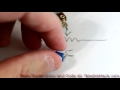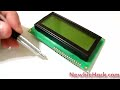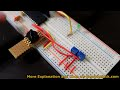50k Through Hole Trimmer PotentiometerIntroduction:

Use this 50K trimmer (variable resistor) to tweak settings on a circuit that will not need to be changed often. These trimmers typically get adjusted only to optimize some needed setting. Use it to resist current, or as a voltage divider.

Description:

Just like a potentiometer, this trimmer has a wiper that adjusts the resistance from one side to the other. There are three leads. The center lead is the wiper and the other leads are the range of resistance.

If you were to measure the resistance from the two range leads, you would get the total 50K resistance in ohms. If you were to measure from the center lead (wiper) to one of the other leads, you would see the proportion of resistance from the position of the wiper to that lead and the other lead would contain the remaining resistance.

50K Trimmer Through Hole

\$0.85
Qty:

Learn about the 50k Through Hole Trimmer PotentiometerThe potentiometer is a device that increases or decreases resistance as a variable resistor. Potentiometers can also be used as a voltage divider. If the potentiometer is to be used as a variable res...Potentiometers are variable resistors. The resistance changes when the wiper is adjusted. The variable resistance is measured from one of the outside leads to the center (wiper) lead. The small vari...The trimmer potentiometers are used to adjust the high and low thresholds for the pressure sensor circuit. The adjustment needs to read between 0 and 33 rather than the ADC result of 0 and 1023, so t... Includes CodeThe code in the ADC channels are updated to reflect the slope intercept formula so the trimmer potentiometers show a range or 0 - 33 rather than the raw ADC result of 0 - 1023. Includes CodeThe code in the ADC channels are updated to reflect the slope intercept formula so the trimmer potentiometers show a range or 0 - 33 rather than the raw ADC result of 0 - 1023. Includes Code